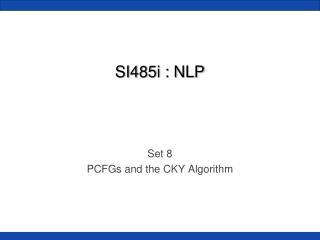Download PresentationSI485i : NLP

# SI485i : NLP - PowerPoint PPT PresentationDownload Presentation## SI485i : NLP

- - - - - - - - - - - - - - - - - - - - - - - - - - - E N D - - - - - - - - - - - - - - - - - - - - - - - - - - -
##### Presentation Transcript

1. SI485i : NLP Set 8 PCFGs and the CKY Algorithm

2. PCFGs • We saw how CFGs can model English (sort of) • ProbabilisticCFGs put weights on the production rules • NP -> DET NN with probability 0.34 • NP -> NN NNwith probability 0.16

3. PCFGs • We still parse sentences and come up with a syntactic derivation tree • But now we can talk about how confident the tree is • P(tree) !

4. Buffalo Example • What is the probability of this tree? • It’s the product of all the inner trees, e.g., P(S->NP VP)

5. PCFG Formalized • G = (T, N, S, R, P) • T is set of terminals • N is set of nonterminals • For NLP, we usually distinguish out a set P ⊂ N of preterminals, which always rewrite as terminals • S is the start symbol (one of the nonterminals) • R is rules/productions of the form X → γ, where X is a nonterminal and γ is a sequence of terminals and nonterminals • P(R) gives the probability of each rule. • A grammar G generates a language model L. Some slides adapted from Chris Manning

6. Some notation • w1n = w1 … wn = the word sequence from 1 to n • wab= the subsequence wa … wb • We’ll write P(Ni → ζj) to mean P(Ni → ζj | Ni ) • Take note, this is a conditional probability. For instance, the sum of all rules headed by an NP must sum to 1! • We’ll want to calculate the best tree T • maxTP(T⇒* wab)

7. Trees and Probabilities • P(t) -- The probability of tree is the product of the probabilities of the rules used to generate it. • P(w1n) -- The probability of the string is the sum of the probabilities of all possible trees that have the string as their yield • P(w1n) = ΣjP(w1n, tj) where tjis a parse of w1n • = Σj P(tj)

8. Example PCFG

9. P(tree) computation

10. Time to Parse • Let’s parse!! • Almost ready… • Trees must be in Chomsky Normal Form first.

11. Chomsky Normal Form • All rules are Z -> X Y or Z -> w • Transforming a grammar to CNF does not change its weak generative capacity. • Remove all unary rules and empties • Transform n-ary rules: VP->V NP PP becomes • VP -> V @VP-V and @VP-V -> NP PP • Why do we do this? Parsing is easier now.

12. Converting into CNF

13. The CKY Algorithm • Cocke-Kasami-Younger (CKY) Dynamic Programming Is back!

14. The CKY Algorithm NP->NN NNS 0.13 p = 0.13 x .0023 x .0014 p = 1.87 x 10^-7 NP->NNP NNS 0.056 p = 0.056 x .001 x .0014 p = 7.84 x 10^-8

15. The CKY Algorithm • What is the runtime? O( ?? ) • Note that each cell must check all pairs of children below it. • Binarizing the CFG rules is a must. The complexity explodes if you do not.

16. Evaluating CKY • How do we know if our parser works? • Count the number of correct labels in your table...the label and the span it dominates • [ label, start, finish ] • Most trees have an error or two! • Count how many spans are correct, wrong, and compute a Precision/Recall ratio.

17. Probabilities? • Where do the probabilities come from? • P( NP -> DT NN ) = ??? • Penn Treebank: a bunch of newspaper articles whose sentences have been manually annotated with full parse trees • P( NP -> DT NN ) = C( NP -> DT NN ) / C( NP )